Get Started »

# Entering Input

In a Wolfram notebook on the desktop or web, just type an input, then press SHIFT+ENTER to compute:

 In:=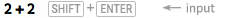X `2 + 2`
 Out=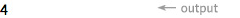In[n] and Out[n] label successive inputs and outputs. The % symbol refers to the most recent output:

 In:=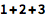⨯ `1 + 2 + 3`
 Out=In:=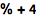⨯ `% + 4`
 Out=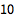After you perform a calculation, the Suggestions Bar will provide options for further computation: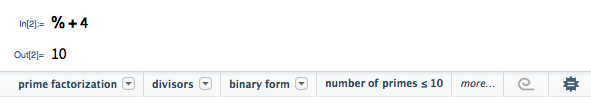Standard symbols work for mathematical operations:

(Use a space or * for multiplication, not the “x” character.)
 In:=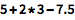⨯ `5 + 2*3 - 7.5`
 Out=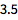Use parentheses (not braces or brackets) to show levels of grouping:

 In:=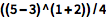⨯ `((5 - 3)^(1 + 2))/4`
 Out=The Wolfram Language has nearly 6,000 built-in functions, covering many areas of mathematics.

Arguments to built-in functions are separated by commas and enclosed in square brackets:

 In:=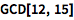⨯ `GCD[12, 15]`
 Out=If you don’t know what function to use, type = at the beginning of a line for natural-language input:

 In:=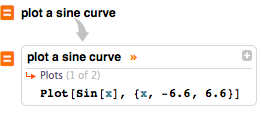X `plot a sine curve`
 Out=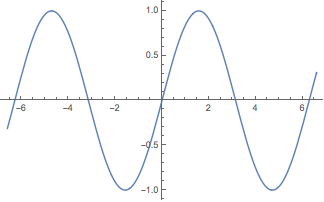Lists represent collections of items and are indicated by { ... }:

 In:=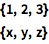⨯ ```{1, 2, 3} {x, y, z}```

Lists are ordered. They can contain numbers, variables, computations or even other lists.

Many operations are applied elementwise:

 In:=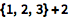⨯ `{1, 2, 3} + 2`
 Out=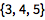Starting at 1, parts of lists can be extracted using [[ ... ]]:

 In:=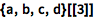⨯ `{a, b, c, d}[]`
 Out=Easily construct lists with functions like Range:

 In:=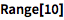⨯ `Range`
 Out=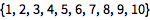QUICK REFERENCE: Managing Computations in Notebooks `»`

QUICK REFERENCE: Free-Form & External Input `»`

QUICK REFERENCE: List Manipulation `»`